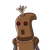# What is the sum of all numbers from 100 to 250, divisible by 6 ?​

What is the sum of all numbers from 100 to 250, divisible by 6 ?​

### 1 thought on “What is the sum of all numbers from 100 to 250, divisible by 6 ?​”

1.5220

Step-by-step explanation:

Lets make an ap as follows: 102, 108, 114… 246.

This will have all numbers divisible by 6 between 100 and 250.

Now,

a = 102

d = 6

tn = 246.

$$tn = a + (n – 1)d \\ 246 = 102 + (n – 1) \times 6 \\ 6n – 6 = 144 \\ 6n = 150 \\ n = 30$$

Thus there are 40 terms. Now let’s find sum.

$$sn = \frac{n}{2} (a + l) \\ = \frac{30}{2} \times (102 + 246) \\ = 15 \times 348 \\ = 5220$$

Thus, the required sum is 5220